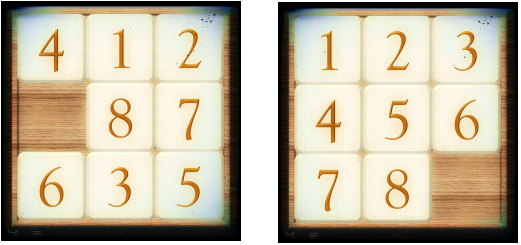# Solving the 8-PuzzleThe 8-puzzle is a sliding puzzle that consists of a frame of numbered square tiles in random order with one tile missing. The object of the puzzle is to place the tiles in order (see the right picture) by making sliding moves that use the empty space.

If one step is defined by sliding exactly one tile to the empty space, at least how many steps are required to solve the puzzle in the left picture?

×